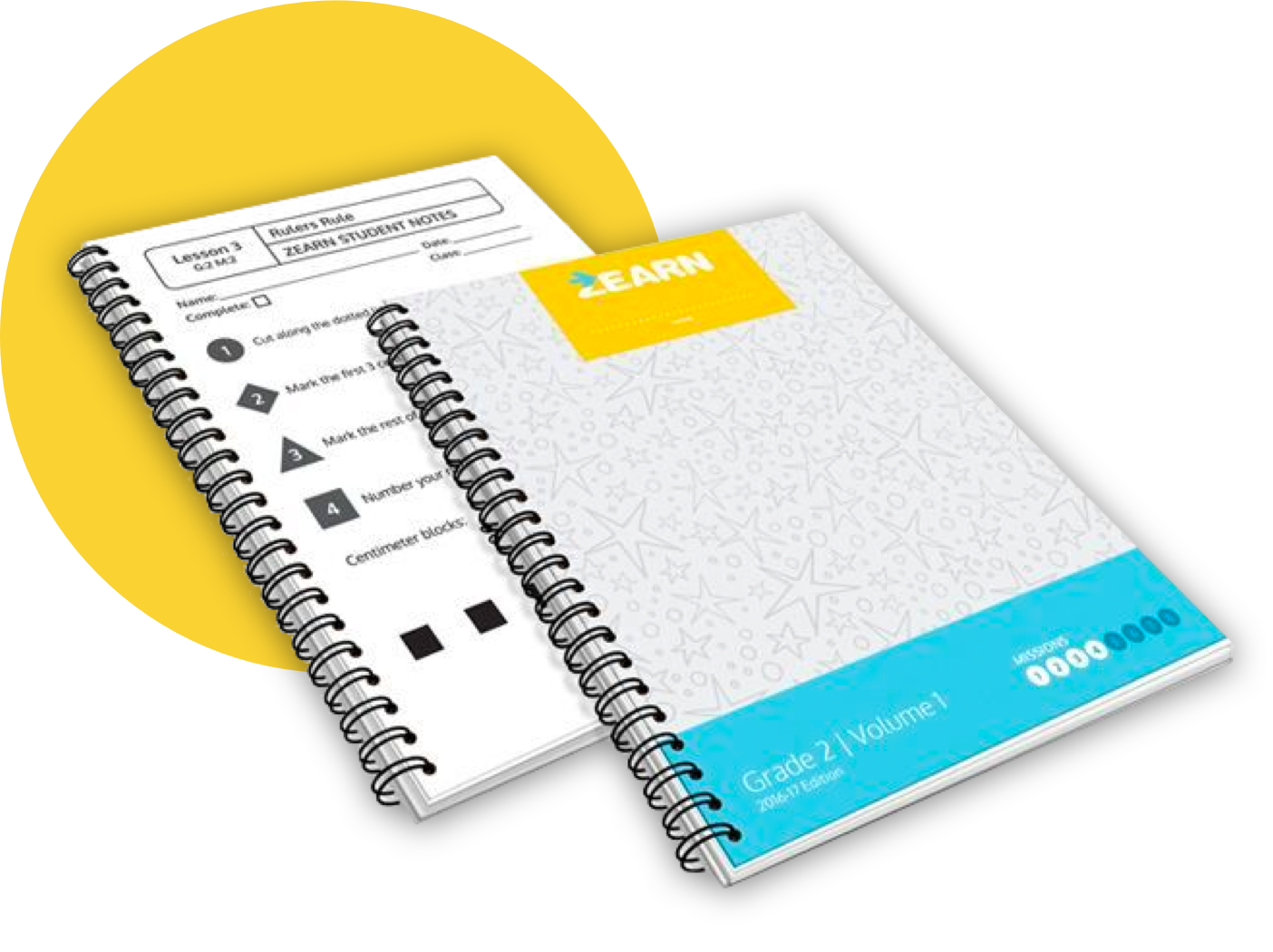# Let's make this summer count.

Our Summer Intensive Series is free for individual teachers and classrooms. Schools and districts can sign up for a paid account to use it with additional features, too.## What you getEach series has daily 30-minute digital lessons that kids complete independently, along with resources for teachers and tutors to use alongside each lesson. All lessons follow the scope and sequence of Eureka Math/EngageNY and can be accessed 24/7 from school, home, or anywhere in between!

### Learning to count with deep understanding

For this year's kindergarten students, entering 1st grade in Fall 2021

Rising 1st grade students practice counting, addition, embedded numbers, and decomposing and composing numbers up to 20 using interactive five frames, ten frames, and number bonds.

K

### Building place value foundations

Rising 2nd grade students work with counting strategies before moving to more sophisticated decomposition and composition strategies. Students then compare, add, and subtract numbers up to 40.

G1

Rising 3rd grade students extend their understanding of place value and decomposing and composing strategies to work through multi-digit subtraction and addition with regrouping.

G2

### Understanding fractions as numbers

Rising 4th grade students work deeply with multiplication and division, and study the concept of fractions as numbers to work with mathematically.

G3

### Understanding fraction equivalence and decimals

Rising 5th grade students learn to multiply and divide big numbers, work deeply with adding and multiplying fractions, and learn about the equivalence between fractions and decimals.

G4

### Mastering operations with whole numbers and fractions